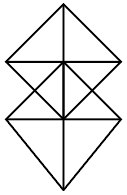Question 19

# How many triangles are there in the following figure ?Solution

If figure is in shape of quadrilateral with two diagonals the number of triangles will be always 8

In this given figure we have two square with diagonals ABCD and EFGH so total 16 triangles are there

Remaining triangles = BIH, CIH, DHJ, CHJ, CIE, CGJ = 6

So, total number of triangles = 8 + 8 + 6 = 22.

• Free SSC Study Material - 18000 Questions
• 230+ SSC previous papers with solutions PDF